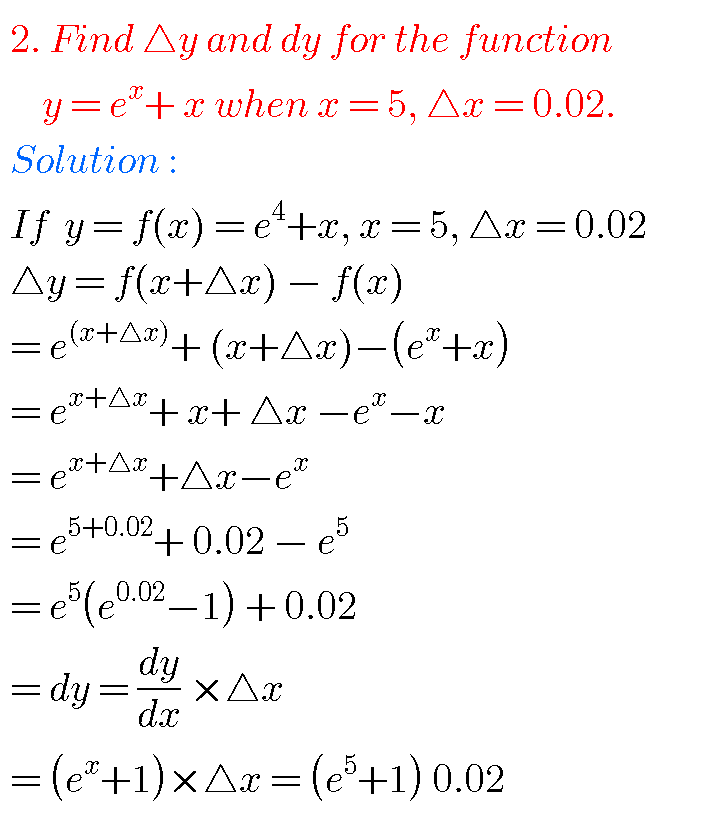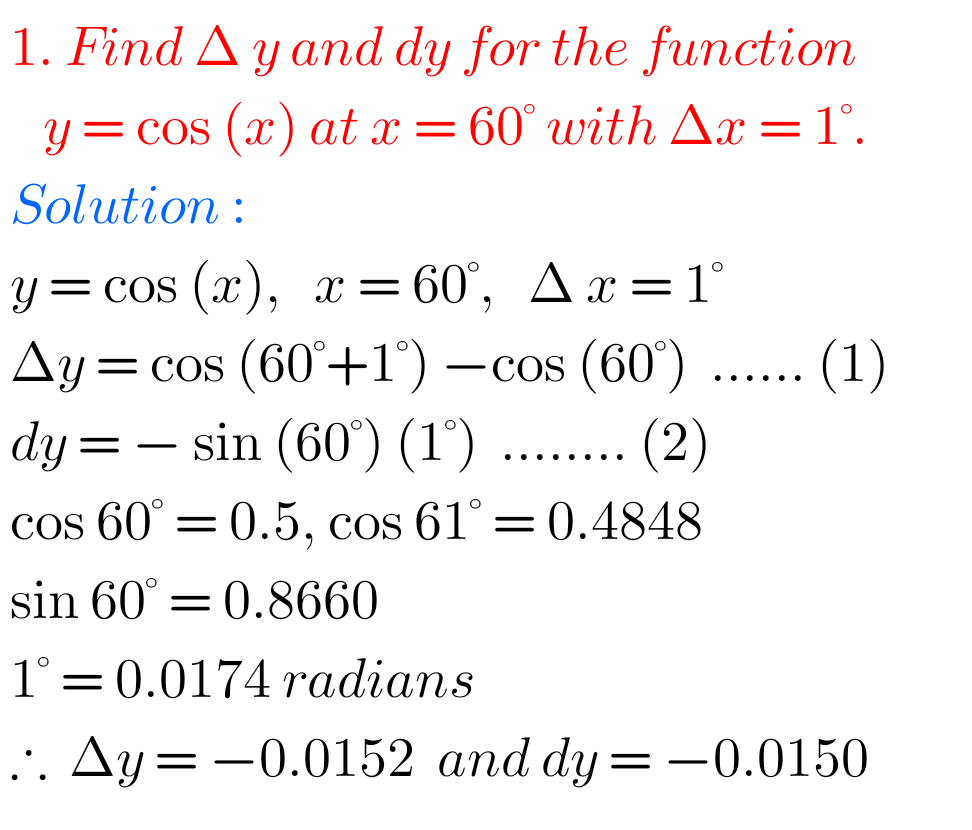# ERRORS AND APPROXIMATION inter first year 1B chapter 10.1 problems with solutions

Mathematics intermediate 1B Errors and Approximation for some problems are available here.

These are very simple to understand.

Study the textbook lesson Applications of Derivatives very well.

Observe the example problems ans solutions given in the textbook.

Textbook exercise solutions links are also given.

Applications of Derivatives

Exercise 10(a)

Exercise 10(b)

Exercise 10(c)

Exercise 10(d)

Exercise 10(e)

Exercise 10(f)

Exercise 10(g)

Exercise 10(h)

You can also see

Inter Maths 1A textbook solutions

Inter Maths 1B textbook solutions

Inter Maths IIA textbook solutions

Inter Maths IIB textbook solutions

M

## CALCULUS – Errors and approximations junior inter maths4

5Note : Observe the solutions and try them in your own methods.You can see the solutions for junior inter maths 1

1. Locus

Straight lines sa

Straight lines la

11. Rate measure

Inter maths trigonometry solutions

Maths real numbers solutions class 10